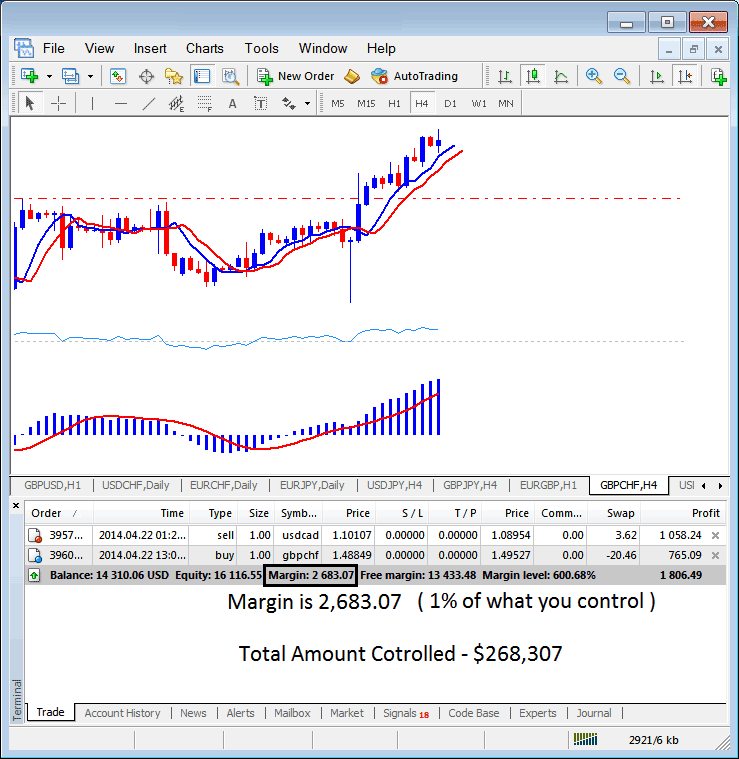This learn indices trading tutorial will explain What is Indices Trading Margin Requirement in stock indexes trading and how it is calculated on the MT4 stock indexes trading platform.

## Margin Level Indices Trading Calculator

If indices trading leverage = 100:1

1,000 / 100,000 * 100= 1%

Margin required = 1%

(1/100 *100= 1%)

(Simplify - your indices trading capital is \$1,000 after stock indices leverage you control \$100,000 - \$1,000 is what percent of \$100,000 - it is 1%) that is your indices trading margin requirement for your stock indexes trading account.

The indices trading margin example on MT4 indices trading Platform below, the set stock indices leverage is 100:1, the indices trading margin which is 1% is \$2683.07, therefore the total amount controlled by the indices trader is: \$268,307 - this is because with this indices trading leverage the indices trader has used little of his money and borrowed the rest, with this set at 100:1, the indices trader is using 1 % of their trading capital, this 1% is \$2683.07, if 1% is \$2683.07 then 100% is \$268,307For 20% margin requirement before closing your stock indexes trades automatically, then your transactions will be closed once your balance gets to \$200

For 50% margin requirement of this level before closing your stock indexes trades automatically, then your transactions will be closed once your balance gets to \$500

If your indices broker sets 100% margin requirement of this level before closing your open positions automatically, then your trades will be closed once your balance gets to \$1,000: Meaning the trade will close out as soon as you execute it because even if you pay 1 pip spread your account balance will get to \$990 and the needed percentage is 100% i.e. 1,000 dollars, therefore your trade orders will immediately get closed.

Most brokers do not set 100% requirement, but there are those brokers that set 100% are not suitable for you at all, choose those set 50% or 20% margin requirements, in fact, those that set at 20% are some of the best because the likely hood they close out your trade is reduced as shown in the examples above.

In the above MetaTrader 4 indices trading screenshot example, the indices trader is using \$2683.07, the total controlled amount is \$268,307, but indices trading account equity is \$16,116.55, therefore used stock indices leverage is (\$268,307 divide by 16,116.55) = 16.64 : 1

16.64 : 1 Used Indices Trading Leverage

The Margin is 600% which is 580% above the required 20% Margin requirement by the stock indexes trading broker.

To know about this margin level which is calculated by your MT4 stock indexes trading platform automatically - The MT4 Indices Trading Platform will display this as "Indices Trading Margin Requirement", This will be displayed as a percentage the higher the percentage the less likely your trades are to get closed.

For Example if - (using a stock indexes trading broker that requires 20% margin requirement)

If stock indices leverage is 100:1 and you transact 10 Mini Lots, equal to \$100,000

\$100,000 dollars divide by 100:1, your used capital is \$1000

Calculation:

= Capital Used * Percentage(100)

= \$1,000/\$1000 * Percentage(100)

Indices Trading Margin Requirement = 100 %

Investor has 80% above the required margin level amount

Using 10:1

If stock indices leverage is 10:1 and you transact 1 Mini Lot, equal to \$10,000

\$10,000 dollars divide by 100:1, your used capital is \$100

Calculation:

= Capital Used * Percentage(100)

= \$1,000/\$100 * Percentage(100)

Indices Trading Margin Requirement = 1000 %

Investor has 980% above the required margin level amount

If stock indices leverage is 1:1 and you transact 0.1 Mini Lot, equal to \$1,000

\$1,000 dollars divide by 100:1, your used capital is \$10

Calculation:

= Capital Used * Percentage(100)

= \$1,000/\$10 * Percentage(100)

Indices Trading Margin Requirement = 10,000 %

Investor has 9800% above the required margin level amount

Start Trading Crypto CFDs with a \$30 Bonus